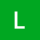Home
IT Knowledge
Inspiration
Languages
EN

# Java - return status code from main()

7 points
Created by:Lennie-S
289

In this short article, we would like to show how to return the status code from the main function in Java.

Quick solution:

``````// put at the end of the main()

System.exit(statusCode);

// e.g.
System.exit(2);``````

## Practical example

`Program.java` file:

``````package example;

public class Program {

public static void main(String[] args) {

System.exit(2); // 0 usually means the program ended successfully
//   other numbers describe error code
// e.g.
//   here we returned error with code 2
}
}``````

Example output:

``Process finished with exit code 2``

Hint: in Bash, we can use exit status from program / command and do some decisions,

e.g.

``````command

if [ \$? = 2 ];
then
echo "error detected";
fi``````

Above code is equivalent to the following c++ / cpp code version:

``````using namespace std;

int main()
{
return 2; // 0 usually means the program ended successfully
//   other numbers describe error code
// e.g.
//   here we returned error with code 2
}``````